Multiply by 20, 25, 50 and 100 – Four Worksheets

Multiplication Table – Multiplication Target Circles – Times Table Target Circles – Times Table Circle Patterns – Multiplication Circles Template

Multiplication Target Circles

Multiply by 20, 25, 50 and 100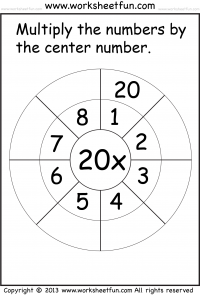Multiply by 20

### Multiply by 50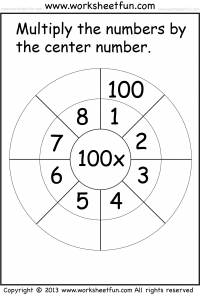Multiplication

Multiplication – Basic Facts

Multiplication – Cubes

Multiplication – Horizontal

Multiplication – Quiz

Multiplication – Test

Multiplication – Vertical

Multiplication Target Circles

Multiplication Word Problems

Multiplication-1 Digit

Multiplication-2 Digit by 2 Digit

Multiplication-3 Digit by 1 Digit

Multiplication-3 Digit by 2 Digit

### New Worksheets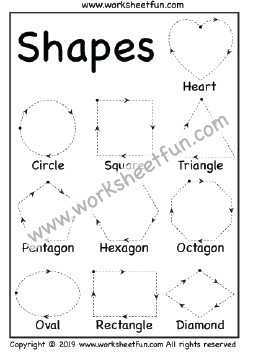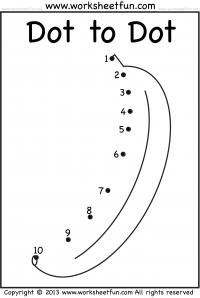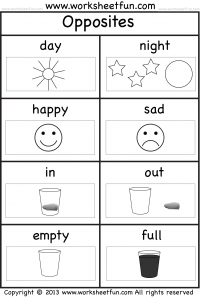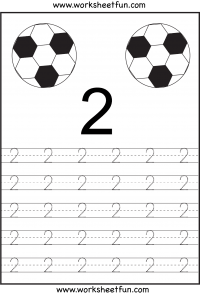### Most Popular Preschool and Kindergarten Worksheets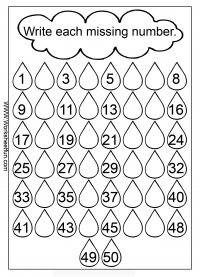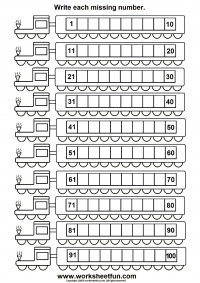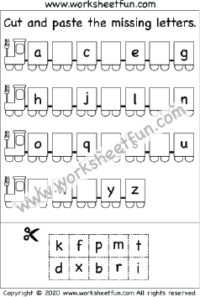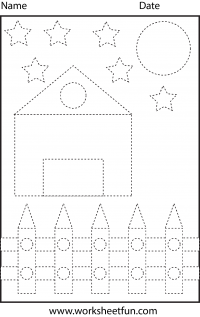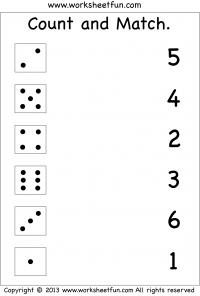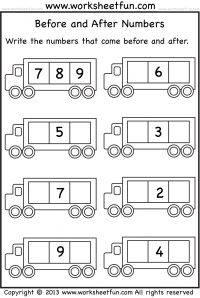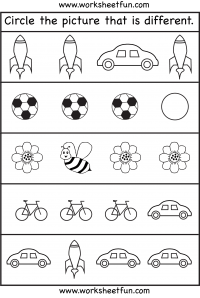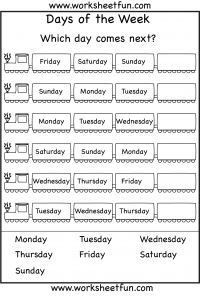#### Kindergarten Worksheets